# Chapter 8 Impulse and Momentum Momentum and Collisions

• Slides: 18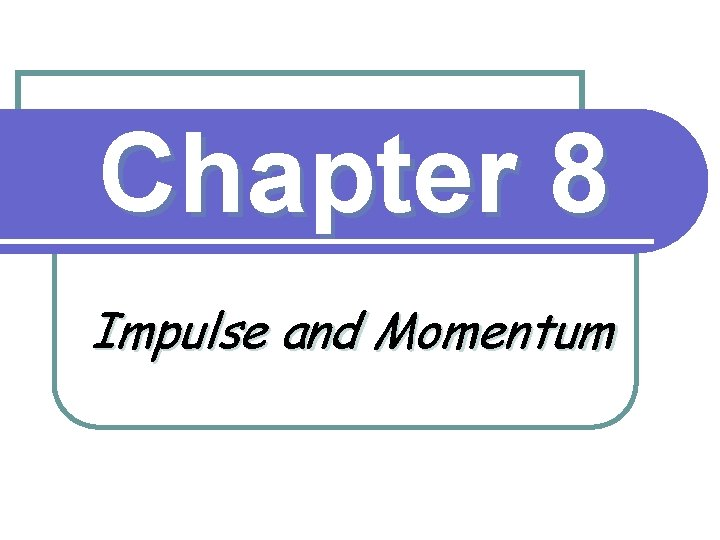Chapter 8 Impulse and Momentum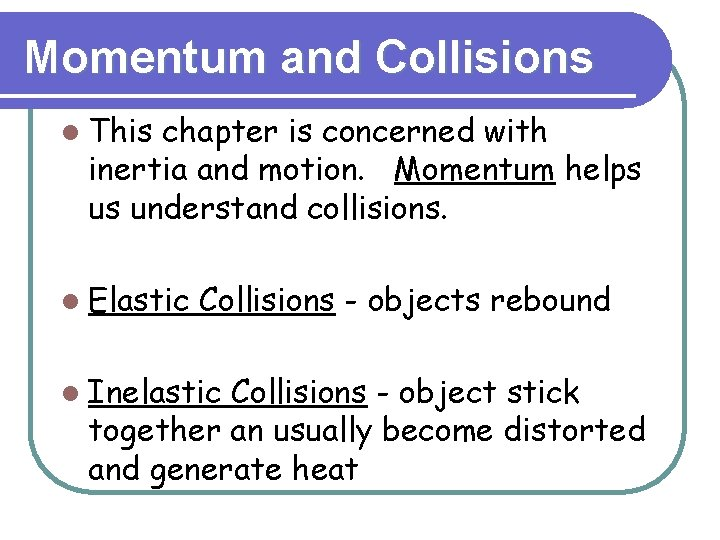Momentum and Collisions l This chapter is concerned with inertia and motion. Momentum helps us understand collisions. l Elastic Collisions - objects rebound l Inelastic Collisions - object stick together an usually become distorted and generate heatMomentum l = mass ´ velocity p = mv l Momentum is a vector quantity.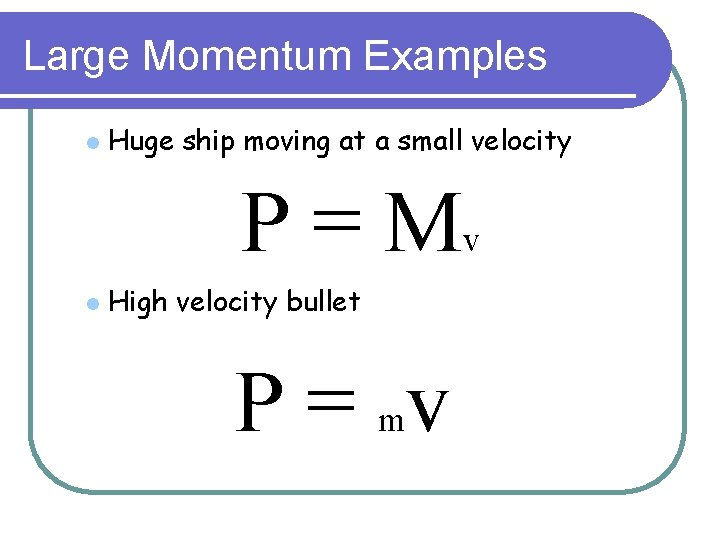Large Momentum Examples l Huge ship moving at a small velocity P = Mv l High velocity bullet P = mv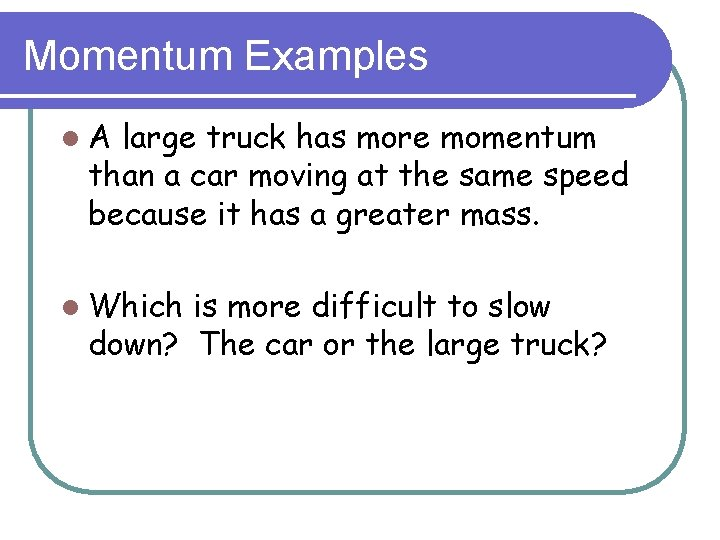Momentum Examples l. A large truck has more momentum than a car moving at the same speed because it has a greater mass. l Which is more difficult to slow down? The car or the large truck?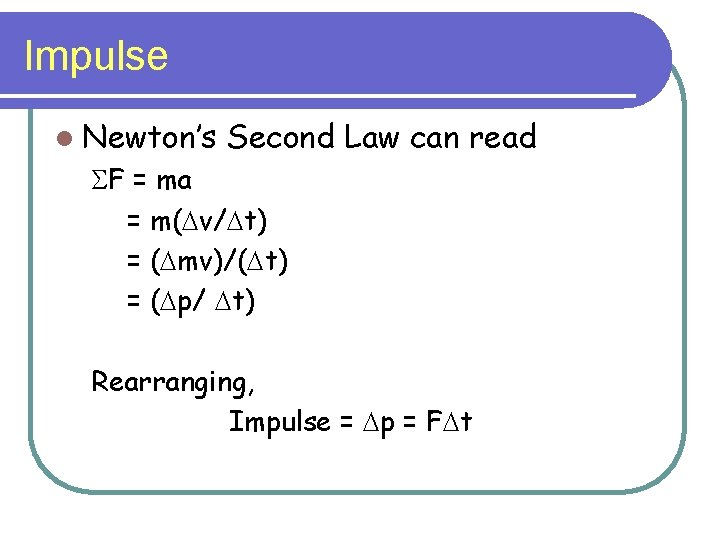Impulse l Newton’s Second Law can read SF = ma = m(Dv/Dt) = (Dmv)/(Dt) = (Dp/ Dt) Rearranging, Impulse = Dp = FDtWhen Force is Limited l Apply l a force for a long time. Examples: Follow through on a golf swing. l Pushing a car. l F Dt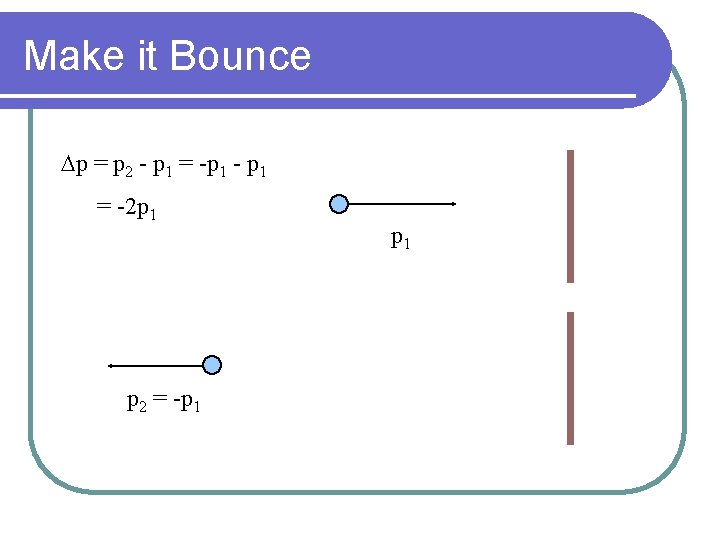Make it Bounce Dp = p 2 - p 1 = -p 1 - p 1 = -2 p 1 p 2 = -p 1Minimize the Force l. Increase Dt l Catching a ball l Bungee jumping F Dt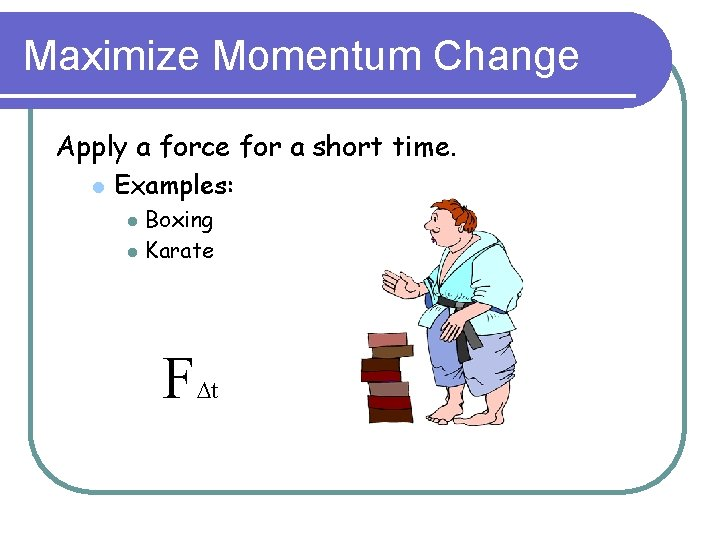Maximize Momentum Change Apply a force for a short time. l Examples: l l Boxing Karate F Dt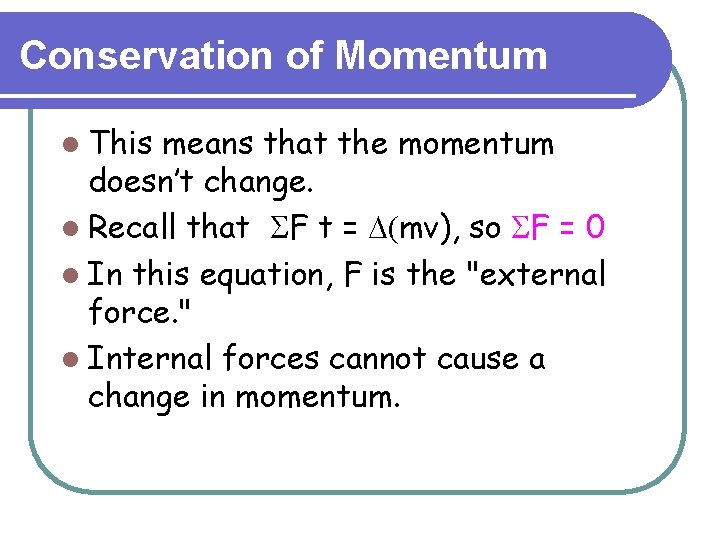Conservation of Momentum l This means that the momentum doesn’t change. l Recall that SF t = D(mv), so SF = 0 l In this equation, F is the "external force. " l Internal forces cannot cause a change in momentum.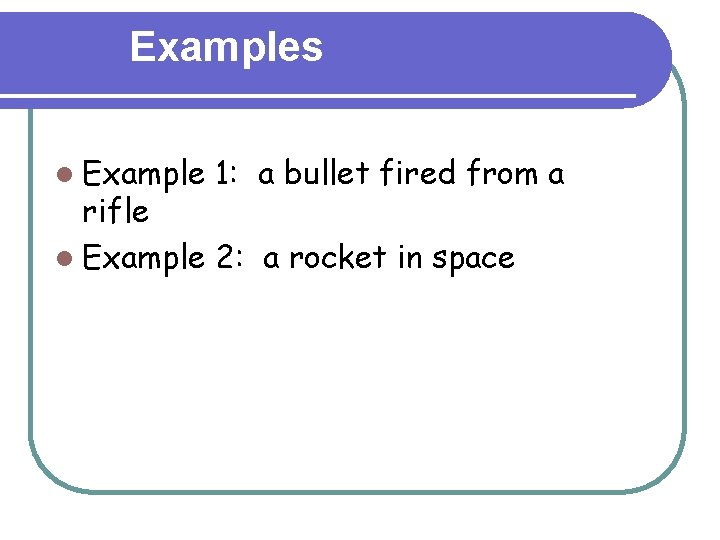Examples l Example 1: a bullet fired from a rifle l Example 2: a rocket in space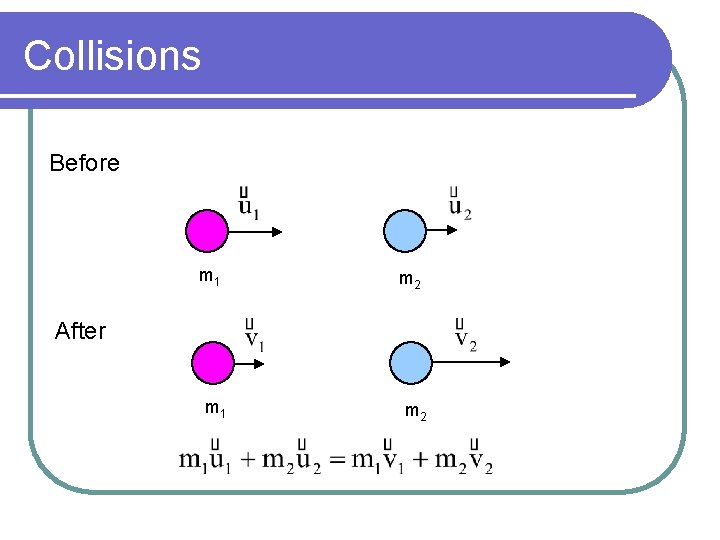Collisions Before m 1 m 2 After m 1 m 2v = 10 v=0 M M Before Collision p = Mv v’ = 5 M M Mv = 2 Mv’ v’ = ½ v After Collision p = 2 Mv’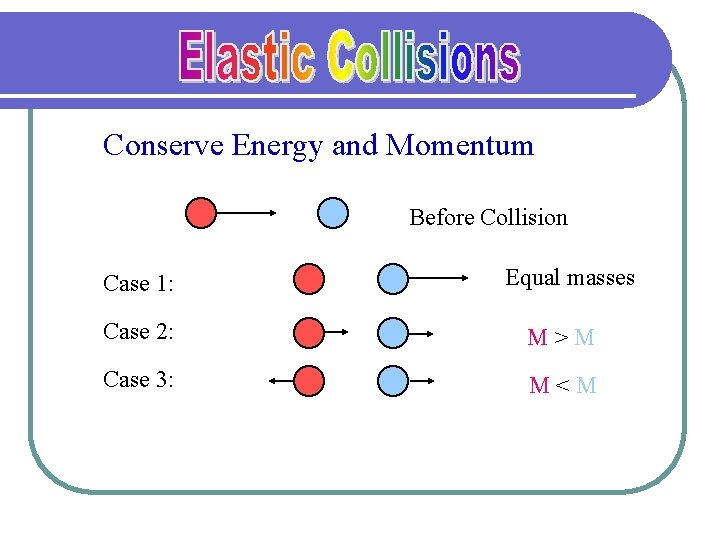Conserve Energy and Momentum Before Collision Case 1: Equal masses Case 2: M>M Case 3: M<MCoefficient of Restitution l For perfectly elastic collisions e = 1. l If the two object stick together, e = 0. l Otherwise 0 < e < 1.Center of Mass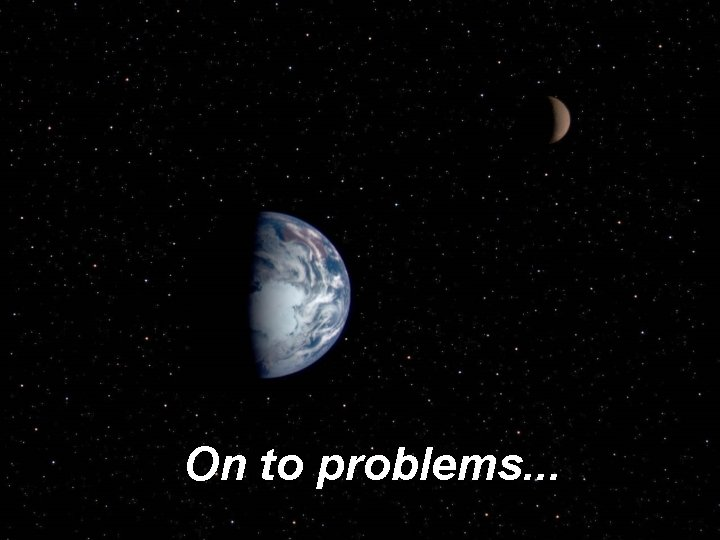On to problems. . .